# Range, mean, median

Ages of 7 employees in an office are given below
32, 42, 30, 32, 33, 23, 32

Find
a) Range
b) Mean
c) Median

a =  19
m =  32
m2 =  32

### Step-by-step explanation:

$m=\frac{23+30+32+32+32+33+42}{7}=32$Did you find an error or inaccuracy? Feel free to write us. Thank you!Tips to related online calculators
Looking for help with calculating arithmetic mean?
Looking for a statistical calculator?
Do you want to convert time units like minutes to seconds?

#### You need to know the following knowledge to solve this word math problem:

We encourage you to watch this tutorial video on this math problem:

## Related math problems and questions:

• The rawThe raw data presented here are the scores (out of 100 marks) of a market survey regarding the acceptability of new product launched by a company for random sample of 50 respondents: 40 45 41 45 45 30 30 8 48 25 26 9 23 24 26 29 8 40 41 42 39 35 18 25 35
• Dataset:Dataset: 35 22 18 54 22 46 28 31 43 22 14 17 25 19 33 14. 1 Group the data into a grouped distribution using 6 classes of equal width. 2. Determine the mean, median, and mode using the raw data. 3. Draw an Ogive curve corresponding to the data and use it
• Here isHere is a data set (n=117) that has been sorted. 10.4 12.2 14.3 15.3 17.1 17.8 18 18.6 19.1 19.9 19.9 20.3 20.6 20.7 20.7 21.2 21.3 22 22.1 22.3 22.8 23 23 23.1 23.5 24.1 24.1 24.4 24.5 24.8 24.9 25.4 25.4 25.5 25.7 25.9 26 26.1 26.2 26.7 26.8 27.5 27.6 2
• Box and whisker plotConstruct a box and whisker plot for the given data. 56, 32, 54, 32, 23, 67, 23, 45, 12, 32, 34, 24, 36, 47, 19, 43
• Speed of Slovakian trainsRudolf decided to take the train from the station 'Ostratice' to 'Horné Ozorovce'. In the train timetables found train Os 5409 : km 0 Chynorany 15:17 5 Ostratice 15:23 15:23 8 Rybany 15:27 15:27 10 Dolné Naštice 15:31 15:31 14 Bánovce nad Bebravou 15:35 1
• TrianglesFind out whether given sizes of the angles can be interior angles of a triangle: a) 23°10',84°30',72°20' b) 90°,41°33',48°37' c) 14°51',90°,75°49' d) 58°58',59°59',60°3'
• Standard deviationFind standard deviation for dataset (grouped data): Age (years) No. Of Persons 0-10 15 10-20 15 20-30 23 30-40 22 40-50 25 50-60 10 60-70 5 70-80 10
• In the 8In the A. P. 36, 39, 42, …, which term is 276?
• SoldierAn experienced soldier digs a good trench in 30 hours. Two soldiers dug the same trench each 40 hours. How many hours will the ditch dig all three together?
• Tropical, mild and arctic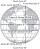How many percent of the Earth's surface lies in the tropical, mild, and arctic range? The border between the ranges is the parallel 23°27' and 66°33'.
• Candles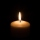Before Christmas, Eva bought two cylindrical candles - red and green. Red was 1 cm longer than green. She lit a red candle on Christmas Day at 5:30 p. M. , lit a green candle at 7:00 p. M. , and left them both on fire until they burned. At 9:30 p. M. , bo
• 5-number summaryGiven the following 5-number summary: 11, 19, 24, 30, 48 which of the statistics cannot be determined?
• Sum of fall dices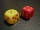What is the probability that the sum of 9 will fall on a roll of two dice? Hint: write down all the pairs that can occur as follows: 11 12 13 14 15. . 21 22 23 24. .. . 31 32. .. . . . . . .. . 66, count them, it's the variable n variable m: 36, 63,. .. .
• The average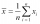The average of one set of 4 numbers is 35. The average of another set of number is 20. The average of the numbers in the two sets is 30. How many numbers are there in the other set?
• Frequency distributionThe following frequency distribution gives the time spent for fill-up at a gas station.  Assume that each value in a class is equal to the midpoint of the class.  Estimate the mean fill-up time for the given data.  Round your answer to the nearest hundred
• Dance ensembles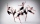4 dance ensembles were dancing at the festival. None had less than 10 and more than 20 members. All dancers from some of the two ensembles were represented in each dance. First, 31 participants were on the stage, then 32, 34, 35, 37, and 38. How many danc
• Mass point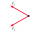Two equal forces of 30 Newtons act on a mass point. Find the magnitude of the resultant force if these forces form an angle of 42°.